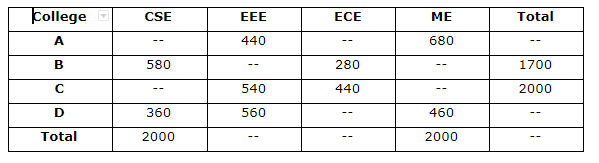# SBI Clerk Prelims 2021 Quantitative Aptitude Questions (Day-53)

Dear Aspirants, Our IBPS Guide team is providing new series of Quantitative Aptitude Questions for SBI Clerk Prelims 2020 so the aspirants can practice it on a daily basis. These questions are framed by our skilled experts after understanding your needs thoroughly. Aspirants can practice these new series questions daily to familiarize with the exact exam pattern and make your preparation effective.

Start Quiz

Table chart

Directions (01-05): Study the following information carefully and answer the questions given below.

The given table shows the number of students in four different colleges in four different departments1) If the ratio of the number of students of CSE from A to C is 21:32, find the number of ME students from C?

A.380

B.360

C.340

D.320

E.None of these

2) Total number of EEE students from all the colleges together is 1900, then what is the number of ME students from B?

A.420

B.460

C.440

D.480

E.None of these

3) If the ratio of the number of ME students from B to C is 24: 19, what is the number of CSE students from C?

A.580

B.600

C.620

D.660

E.None of these

4) If the total number of students from A is equal to the total number of ME students from all the colleges together. The number of ECE students from C is approximately what percent of the total number of students from A all the departments together?

A.18%

B.20%

C.22%

D.24%

E.None of these

5) If the average number of ECE students from all the colleges together is 430 and the number of ECE students from D is equal to the number of ME students from A. What is the ratio of the number of ECE students from A to the number of CSE students from D?

A.7:8

B.8:9

C.5:6

D.4:5

E.None of these

Approximations

Directions (06-10): What approximate value should come in the place of (?) in the following questions?

6) 11.112% of 17.904 + 54.902 % of 299.945 – 149.921 % of 59.912 = ?

A.66

B.77

C.88

D.44

E.55

7) 119.941% of 300.12 + √5185.102 =? * √256.123

A.23

B.27

C.31

D.35

E.40

8) 239.987 ÷ 5.942 + √529.12 * 6.120 =? + 117.918

A.40

B.50

C.60

D.70

E.80

9) 19.896 * 3.902 + √290 * 1.905 + 45.190 % of 79.917=?

A.120

B.140

C.130

D.150

E.160

10) 49.925 % of 77.879 + ? % of 37.012 = 160.934 * 2.903

A.1100

B.1300

C.1000

D.1200

E.1400

Total number of CSE students =2000

Number of CSE students from A and C = 2000 – 580 – 360 = 1060

Number of CSE students from C = 32/53 * 1060 = 640

Number of ME students from C = 2000 – 640 – 540 – 440 = 380

Number of EEE students from B = 1900 – 440 – 540 – 560 = 360

Number of ME students from B = 1700 – 580 – 360 – 280 = 480

Number of ME students from B and C = 2000 – 680 – 460 = 860

Number of ME students from C = 19/43 * 860 = 380

Number of CSE students from C = 2000 – 540 – 440 – 380 = 640

Required percentage = 440/2000 * 100 = 22%

Total number of ECE students = 430 * 4 = 1720

Number of ECE students from D = 680

Number of ECE students from A = 1720 – 280 – 440 – 680 = 320

Required ratio = 320: 360 = 8: 9

11.112% of 17.904 + 54.902 % of 299.945 – 149.921 % of 59.912 = ?

100/9 * 18 + 55/100 * 300 – 150/100 * 60 = ?

2 + 165 – 90 = ?

? = 77

119.941% of 300.12 + √5185.102 =? * √256.123

120/100 * 300 + √5184 = ? * √256

360 + 72 =? * 16

? = 27

239.987 ÷ 5.942 + √529.12 * 6.120 = ? + 117.918

240/6 + √529 * 6 = ? + 118

40 + 138 = ? + 118

? = 60

19.896 * 3.902 + √290 * 1.905 + 45.190 % of 79.917 = ?

20 * 4 + √289 * 2 + 45/100 * 80 = ?

80 + 34 + 36 = ?

? = 150

49.925 % of 77.879 + ? % of 37.012 = 160.934 * 2.903

50/100 * 78 + ? /100 * 37 = 161 * 3

39 + ? % 37 = 483

? = 1200

 Check Here to View SBI Clerk Prelims 2021 Quantitative Aptitude Questions Day – 52 Day – 51 Day – 50